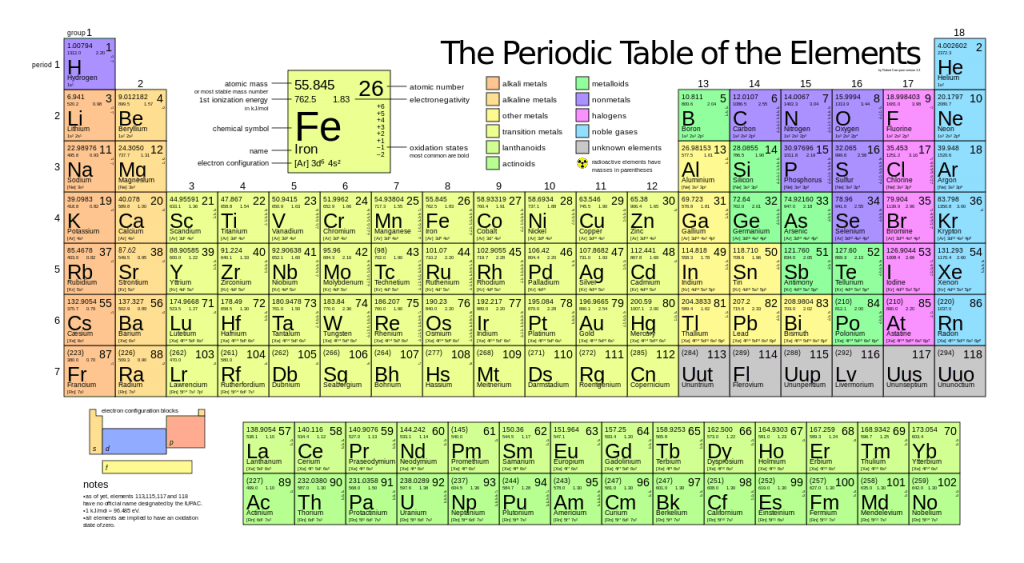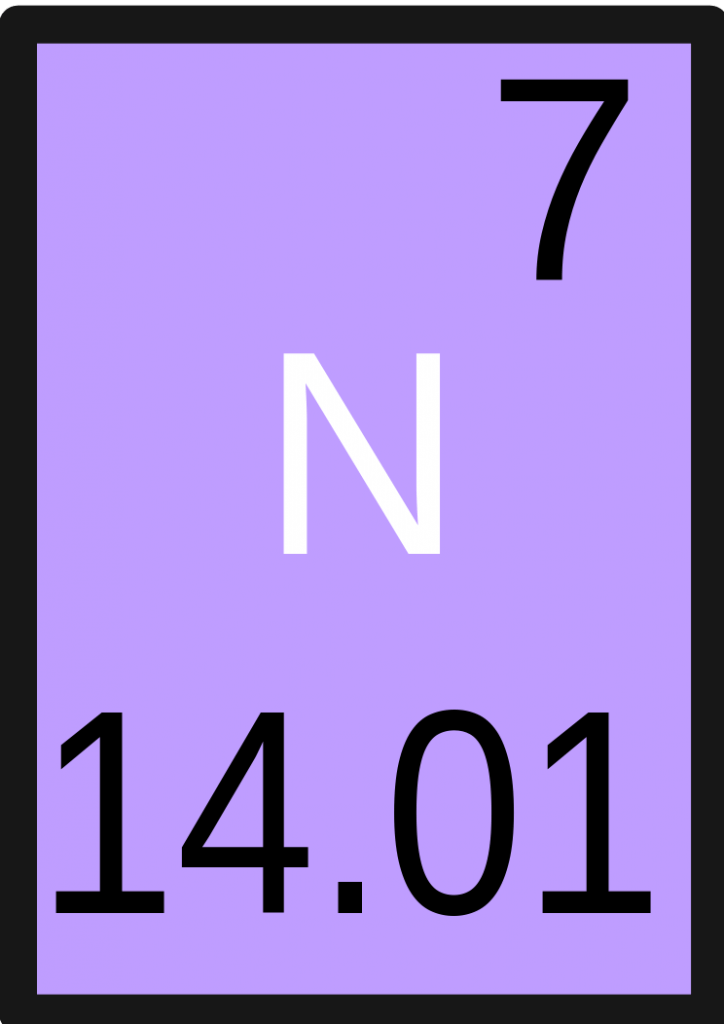# Difference Between Mass Number and Atomic Mass

## Main Difference – Mass Number vs Atomic Mass

Both Mass Number and Atomic Mass are very closely related to each other and indicates the weight of elements. They differ in the way they are defined. The main difference between mass number and atomic mass is that mass number deals with individual atoms taken into consideration separately whereas atomic mass deals with the weighted average of the element including its isotopes.

## What is Mass Number

As mentioned above, the mass number is associated with the weight of a single atom under consideration. An atom is made up of a nucleus and orbitals around it. These orbitals exist in free space and accommodate electrons. However, the weight of the electrons is negligible when compared to the nuclear core. Therefore, the weight of the electrons does not contribute to the weight of an atom/element. The nucleus is made up of protons and neutrons. Both neutrons and protons are almost the same in weight but changes very slightly.  Protons are positively charged and the neutrons are neutral in charge. Therefore, the nucleus remains positively charged. However, the atom as a whole is considered as neutral entities as the positive charge from the protons are neutralized by the negative charge of the electrons. The number of electrons in an atom equals the number of protons.

The elements in the periodic table are arranged according to an increasing mass number. The mass number is symbolically represented in the upper left-hand corner when an element is labeled. The mass number differs from the atomic number as it represents only the number of protons or electrons in an element. And the atomic number is usually written on the bottom left-hand corner of an element.## What is Atomic Mass

This term is very closely related to the mass number. It actually means the same thing literally, however, in this case, the isotopic forms of each element are also accounted. Elements can exist in various forms in nature. These different forms are generally known as isotopes, and they have the same identity as the most abundant/stable form the element. Therefore, isotopes have the same atomic number, but they have different mass numbers. It can be concluded that isotopes carry the same amount of protons and electrons; it is only the number of neutrons that differ. Therefore, what differs between them is the weight.

When taking each isotopic form into consideration, the mass of an elemental form can be expressed as an average value, where the individual masses of each isotopic form are averaged out. This is referred as the ‘Atomic Mass’ of an element. Therefore the atomic mass has almost the same numerical value as the mass number, with only a change of few decimal values. Each number is used for its purposes of convenience depending on the context of use.## Difference Between Mass Number and Atomic Mass

### Definition

The Mass Number is the weight of the nucleus of an atom.

The Atomic Mass is the average weight of an element form.

### Symbol

The Mass Number is written on the upper left-hand corner of an elemental expression.

The Atomic Mass is not symbolized as such.

### Consideration of Isotopes

Mass Number doesn’t take the existence of isotopes into consideration, as it relates to the weight of each atom separately.

The Atomic Mass relates to the average weight of an elemental form and, therefore, it takes into consideration the existence of isotopes and their abundance.

### Numerical Value

The Mass Number is a simplified number, usually without decimal places.

The Atomic Mass deals with several decimal values as it is a weighted average.

### Ease of Use

The Mass Number is easier to use for general purposes.

The Atomic Mass is helpful when performing specific calculations.Image Courtesy:

“Periodic table large” by 2012rc – Own workNotes and font fixed: The Photographer. (CC BY 3.0) via Wikimedia Commons

“Nitrogen” by me – Image:Nitrogen.gif. (CC BY-SA 2.5) via Wikimedia Commons